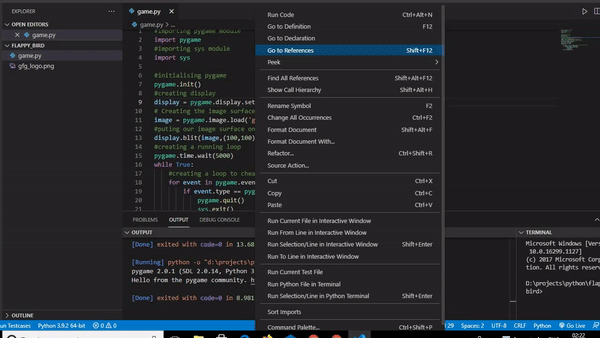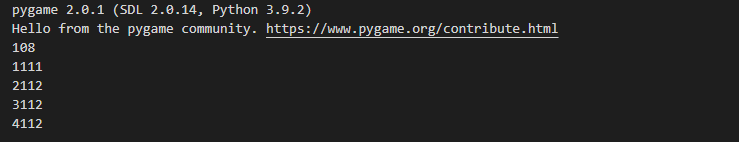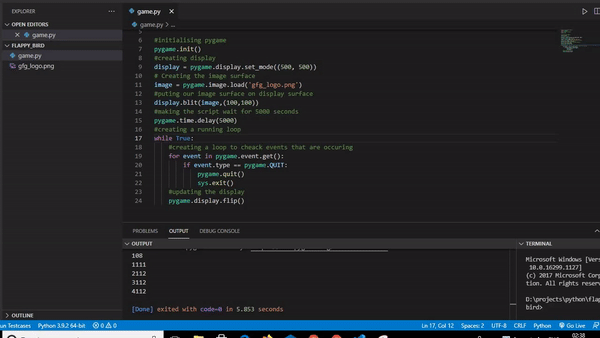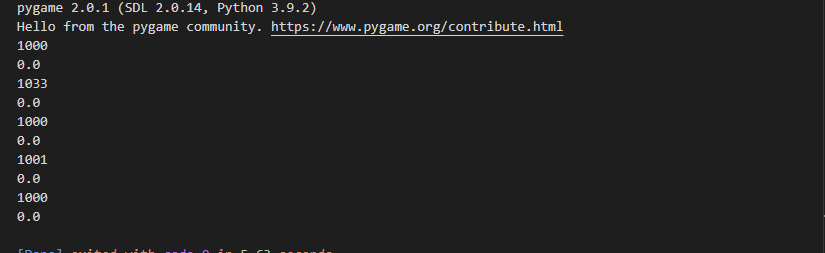# Pygame – Time

• Last Updated : 25 Oct, 2021

While using pygame we sometimes need to perform certain operations that include the usage of time. Like finding how much time our program has been running, pausing the program for an amount of time, etc. For operations of this kind, we need to use the time methods of pygame. In this article, we will be discussing the various methods that can be used for performing these operations.

The function that we will discuss are:-

• pygame.time.wait
• pygame.time.get_ticks
• pygame.time.delay
• pygame.time.Clock

## pygame.time.wait

This function is used to pause the running of the program for few seconds. it takes time in milliseconds as parameter. For example to demonstrate this function we will write a simple program to make geeksforgeeks logo appear on screen only after 5 seconds. The code for this will be:

## Python

 `# importing pygame module` `import` `pygame`   `# importing sys module` `import` `sys`   `# initialising pygame` `pygame.init()`   `# creating display` `display ``=` `pygame.display.set_mode((``500``, ``500``))`   `# Creating the image surface` `image ``=` `pygame.image.load(``'gfg_logo.png'``)`   `# putting our image surface on display surface` `display.blit(image,(``100``,``100``))`   `# making the script wait for 5000 seconds` `pygame.time.wait(``5000``)`   `# creating a running loop` `while` `True``:` `  `  `      ``# creating a loop to check events that are occurring` `    ``for` `event ``in` `pygame.event.get():` `        ``if` `event.``type` `=``=` `pygame.QUIT:` `            ``pygame.quit()` `            ``sys.exit()` `    ``# updating the display` `    ``pygame.display.flip()`

Output:The output of this will be that the script will wait for 5 seconds and then update the display to show geeksforgeeks logo.

It is slightly less accurate than pygame.time.delay which we will discuss later in this article because it uses sleeping but the other one uses the processor.

## pygame.time.get_ticks

This function gives a time which has been running in milliseconds. For example, if we want to write a simple code to demonstrate this example, it can be:

## Python

 `# importing pygame module` `import` `pygame`   `# initialising pygame` `pygame.init()`   `# creating a variable ` `i``=``0` `while` `i<``5``:` `     `  `    ``# storing the time in ticks variable` `    ``ticks``=``pygame.time.get_ticks()` `    `  `    ``# printing the variable ticks` `    ``print``(ticks)` `    `  `    ``# increasing the value of i by 1` `    ``i``=``i``+``1` `    `  `    ``# pausing the script for 1 second` `    ``pygame.time.wait(``1000``)`

p;
Output:The time is printed for every iteration, including the time for which we paused the script in every iteration.

## pygame.time.delay

This function works the same as pygame.time.wait function the difference is that this function will use the processor (rather than sleeping) in order to make the delay more accurate. The sample code can be written the same as pygame.time.wait function by just replacing the name:

## Python

 `# importing pygame module` `import` `pygame`   `# importing sys module` `import` `sys`   `# initialising pygame` `pygame.init()`   `# creating display` `display ``=` `pygame.display.set_mode((``500``, ``500``))`   `# Creating the image surface` `image ``=` `pygame.image.load(``'gfg_logo.png'``)`   `# putting our image surface on display surface` `display.blit(image,(``100``,``100``))`   `# making the script wait for 5000 seconds` `pygame.time.delay(``5000``)`   `# creating a running loop` `while` `True``:` `  `  `      ``# creating a loop to check events that are occurring` `    ``for` `event ``in` `pygame.event.get():` `        ``if` `event.``type` `=``=` `pygame.QUIT:` `            ``pygame.quit()` `            ``sys.exit()` `    ``# updating the display` `    ``pygame.display.flip()`

Output:## pygame.time.Clock

This function is used to create a clock object which can be used to keep track of time. The various methods of clock object are below:

tick():This method should be called once per frame. It will compute how many milliseconds have passed since the previous call. If you pass the optional framerate argument the function will delay to keep the game running slower than the given ticks per second. For example if we pass 10 as argument the program will never run at more than 10 frames per second.

get_time():It is used to obtain a number of milliseconds used between two tick().

get_fps():it gives information regarding the clock frame rate. it returns the output in floating-point value.

A simple Program to demonstrate this function could be:

## Python

 `# importing the pygame module` `import` `pygame`   `# initialising the pygame` `pygame.init()`   `# declaring a variable i with value 0` `i``=``0`   `# creating a clock object` `clock``=``pygame.time.Clock()`   `# creating a loop for 5 iterations` `while` `i<``5``:` `      `  `    ``# setting fps of program to max 1 per second` `    ``clock.tick(``1``)` `    `  `    ``# printing time used in the previous tick` `    ``print``(clock.get_time())` `    `  `    ``# printing compute the clock framerate` `    ``print``(clock.get_fps())` `    ``i``=``i``+``1`

Output:As we have passed 1 in the tick method, it sets maximum fps to 1. Which results in time between each frame nearing 1000 milliseconds.

My Personal Notes arrow_drop_up
Recommended Articles
Page :